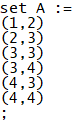# Printing Coordinates to a csv File

I would like to extract coordinates to a .csv file with the brackets and comma delimiter format (x,y).

I have a 4×4 matrix written as a list (network1) and need to identify the coordinates where a 1 occurs to then export these coordinates to a .csv file.

The code below was suggested by another user which works great for a different set of data, although I need to adjust this a bit further to suit this format.

I expect there is only a small modification needed in the existing code which is below.

``````import numpy as np
import pandas as pd

network1 = [0,1,0,0,0,0,1,0,0,0,1,1,0,0,1,1]
network1_matrix = np.array(network1).reshape(4, 4)

coordinates = np.transpose(np.where(network1_matrix == 1))

result_df = pd.DataFrame({'1': coordinates[:, 0] + 1, '2': coordinates[:, 1] + 1})
result_df = result_df.append({'1': ';', '2': ''}, ignore_index=True)
result_df.columns = ['set A :=', '']

result_df.to_csv('result.csv', sep=' ', index=False)
``````

This produces an output as follows (I have included results from a text file for greater clarity):For this specific output, I need it in the following format:I would greatly appreciate the assistance to complete the following as per the second image:

1. Print set A := to the .csv file without the quotation marks (" ") around it.
2. Print the coordinates with the brackets and only delimited by a comma.

Thanks a lot for the help!